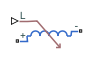# Variable Inductor

Linear time-varying inductor

•Libraries:
Simscape / Electrical / Passive

## Description

The Variable Inductor block represents a linear time-varying inductor. The block provides two options for the relationship between the voltage v across the device and the current through the inductor i when the inductance at port L is L. The Equation parameter determines which of the following equations the block uses:

• $v=\frac{dL}{dt}i+L\frac{di}{dt}$

Use the preceding equation when the inductance is defined as the ratio of the magnetic flux Ф to the steady-state current:

`$L\left(i\right)=\frac{\Phi \left(i\right)}{i}$`

• $v=L\frac{di}{dt}$

Use the preceding equation when the inductance is defined as the local gradient of the flux-current curve for a given current:

`$L\left(i\right)=\frac{d\Phi \left(i\right)}{di}$`

The block includes a conductance in parallel with the variable inductor. You can use the conductor to represent the total insulation conductance of the inductor. You may need to use the conductor to prevent numerical issues for some circuit topologies, such as where a Variable Inductor block is connected in series with another inductor block that does not have a parallel conductance.

## Ports

### Input

expand all

Physical signal input port associated with the inductance. L must be finite, greater than zero, and continuous (no step changes).

### Conserving

expand all

Electrical conserving port associated with the inductor positive voltage.

Electrical conserving port associated with the inductor negative voltage.

## Parameters

expand all

Select one of the following options for block inductance:

• `V = L*dI/dt + dL/dt*I` — This equation assumes the inductance is defined as the ratio of the magnetic flux to the steady-state current. This option is the default.

• `V = L*dI/dt` — This equation assumes the inductance is defined as the local gradient of the flux-current curve for a given current.

The lower limit on the value of the signal at port L. This limit prevents the signal from reaching a value that has no physical meaning.

The value of the conductance placed in parallel with the variable inductor.

The output current at the start of the simulation.

#### Dependencies

This parameter is visible only when you select ```V = L*dI/dt``` for the Equation parameter.

The magnetic flux at the start of the simulation.

#### Dependencies

This parameter is visible only when you select ```V = L*dI/dt + dL/dt*I``` for the Equation parameter.

## Version History

Introduced in R2008a